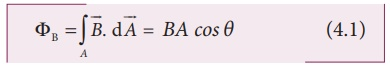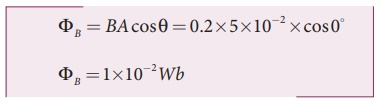Home | | Physics 12th Std | Magnetic Flux

# Magnetic Flux

The magnetic flux through an area A in a magnetic field is defined as the number of magnetic field lines passing through that area normally.

Magnetic Flux (Î¦B)

The magnetic flux through an area A in a magnetic field is defined as the number of magnetic field lines passing through that area normally and is given by the equation (Figure 4.1(a)).where the integral is taken over the area A and Î¸ is the angle between the direction of the magnetic field and the outward normal to the area.If the magnetic fieldis uniform over the area A and is perpendicular to the area as shown in Figure 4.1(b), then the above equation becomesThe SI unit of magnetic flux is T m2. It is also measured in weber or Wb.

1 Wb = 1 T m2

EXAMPLE 4.1

A circular antenna of area 3 m2 is installed at a place in Madurai. The plane of the area of antenna is inclined at 47Âş with the direction of Earthâ€™s magnetic field. If the magnitude of Earthâ€™s field at that place is 40773.9 nT find the magnetic flux linked with the antenna.

Solution

B = 40773.9 nT; Î¸ = 90Âş  â€“ 47Âş  = 43Â°;

A = 3m2

We know that  Î¦B = BAcosÎ¸EXAMPLE 4.2

A circular loop of area 5 x 10-2m2 rotates in a uniform magnetic field of 0.2 T. If the loop rotates about its diameter which is perpendicular to the magnetic field as shown in figure. Find the magnetic flux linked with the loop when its plane is (i) normal to the field (ii) inclined 60o to the field and (iii) parallel to the field.Solution

A = 5 Â´ 10-2 m2; B = 0.2 T

(i) Î¸ = 0Â°Tags : Definition, Formula, Solved Example Problems | Electromagnetic Induction , 12th Physics : Electromagnetic Induction and Alternating Current
Study Material, Lecturing Notes, Assignment, Reference, Wiki description explanation, brief detail
12th Physics : Electromagnetic Induction and Alternating Current : Magnetic Flux | Definition, Formula, Solved Example Problems | Electromagnetic Induction